Begtostudy(白途思)'s Professional Technology Blog

1.1 总体与样本

1.1.1简单随机样本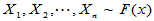，i.i.d，独立同分布。无限总体抽样。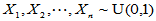，均匀分布样本

n=10;x=rand(1,n)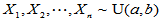n=10;a=-1;b=3;x=rand(1,n);x=(b-a)*x+a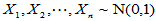，正态分布样本

n=10;x=randn(1,n)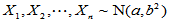mu=80.2;sigma=7.6;m=1;n=10;
x=normrnd(mu,sigma,m,n)

x=betarnd(a,b,m,n) 参数为a,bbeta分布；

x=binornd(N,p,m,n) 参数为N,p的二项分布；

x=chi2rnd(N,m,n) 自由度为N分布；

x=exprnd(mu,m,n) 总体期望为mu的指数分布；

x=frnd(n1,n2,m,n) 自由度为n1n2F分布；

x=gamrnd(a,b,m,n) 参数为a,b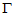分布；

x=lognrnd(mu,sigma,m,n) 参数为musigma的对数正态分布；

x=poissrnd(mu,m,n) 总体均值为muPoisson分布；

x=trnd(N,m,n) 自由度为NT分布；

Matlab统计工具箱中还有一些其它分布，不再一一列举。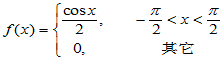n=1000;

x=zeros(1,n);

k=0;

while k<n

a=rand*pi-pi/2;

b=rand/2;

if b<(cos(a)/2)

k=k+1;

x(k)=a;

end

end

hist(x,-pi/2:0.2:pi/2)

1.1.2有限总体的无放回样本

r=randperm(N)

x=X(r(1:n))

1.2 统计量

1.2.1样本k阶矩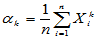为样本k原点矩，对于已经赋值的正整数k,可以用如下命令得到

a(k)=mean(X.^k)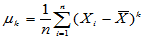为样本k中心矩，对于已经赋值的正整数k,可以用如下命令得到

mu(k)=mean((X-mean(X)).^k)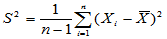1.2.2顺序统计量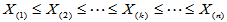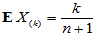Y=sort(X)

1.2.3经验分布函数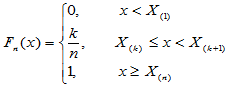1-1）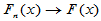a.s.

Y=chi2rnd(5,1,1000);[F,X]=ecdf(Y);

plot(X,F)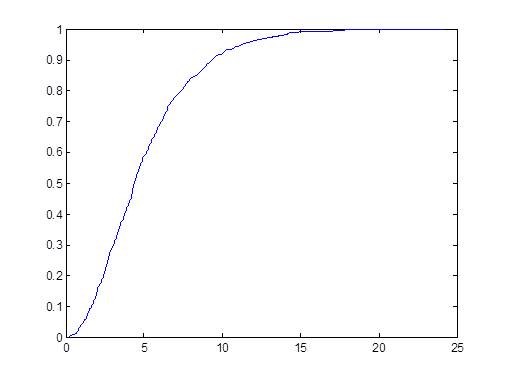function y=myfn(t,x)

N=length(x); x=sort(x);

[m,n]=size(t); y=zeros(m,n);

for i=1:m

for j=1:n

s=0;

for k=1:N

if t(i,j)>=x(k)

s=s+1;

end

end

y(i,j)=s/N;

end

end

1.3 三个常用分布

1.3.1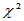分布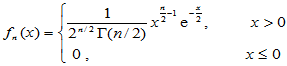（1-2）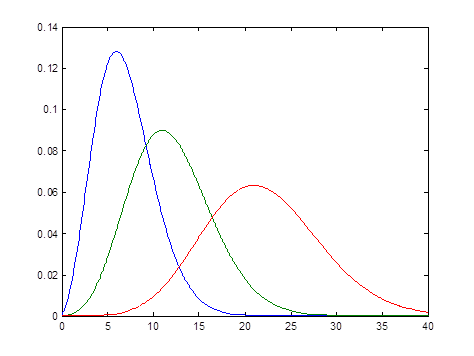（1）期望与方差：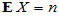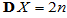（2）来源：若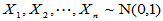独立同分布，则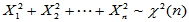（3）可加性：若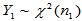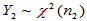，且两者独立，则有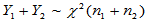(4)重要结论：若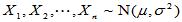，则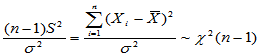1.3.2 t分布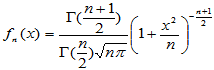（1-3）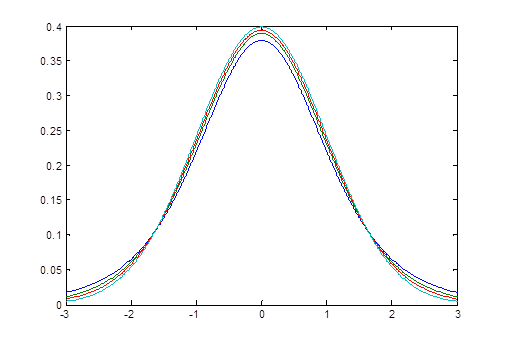（1）密度函数特点：与标准正态分布类似，方差较大。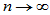时，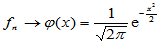（标准正态分布密度函数）

x=-3:0.01:3; y5=tpdf(x,5);y10=tpdf(x,10);

y20=tpdf(x,20);y=normpdf(x);

plot(x,y5,x,y10,x,y20,x,y)

（2）来源：设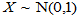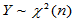，且两者独立，则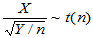（3）重要结论：设，则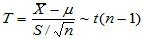1.3.3 F分布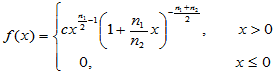（1-4）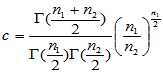（1）密度函数特点：在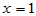附近密度函数取值较大，为单峰非对称的。当两个自由度都很大时，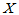取值以较大概率集中在附近。以下Matlab命令画出了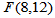的密度函数。

x=0:0.01:3;y=fpdf(x,8,12);plot(x,y);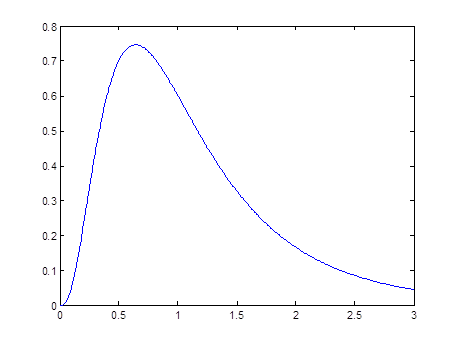（2）来源：设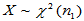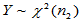，且两者独立，则（3）重要结论：设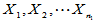为来自总体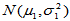的简单随机样本，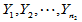为来自总体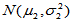的简单随机样本，且两者独立。又设两个样本方差分别为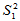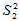，则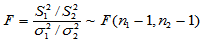Email：begtostudy#gmail.com